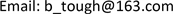1中车株洲电力机车有限公司，湖南 株洲

2同济大学磁浮交通工程技术研究中心，上海1. 引言

2. 主电路原理分析

1) 当 0 < t < t 1 时：

R i 0 ( t ) + L d i 0 ( t ) d t = − U d (1)

d i 0 ( t ) d t + R L i 0 ( t ) + U d L = 0 (2)

i 0 ( t ) = U d R ( e − R t L − 1 ) + I 01 e − R t L (3)

I 02 = U d R ( e − R t 1 L − 1 ) + I 01 e − R t 1 L (4)

2) 当 t 1 < t < T 时

R i 0 ( t ) + L d i 0 ( t ) d t = U d (5)

d i 0 ( t ) d t + R L i 0 ( t ) − U d L = 0 (6)

i 0 ( t ) = U d R ( 1 − e − R ( t − t 1 ) L ) + I 02 e − R ( t − t 1 ) L (7)

3) 当 t = T 时， i 0 ( T ) = I 01 ，代入上式(7)可得：

I 01 = U d R ( 1 − e − R ( T − t 1 ) L ) + I 02 e − R ( T − t 1 ) L (8)

I 01 = U d ( e − R T L − 2 e − R ( T − t 1 ) L + 1 ) R ( 1 − e − R T L ) (9)

I 02 = U d ( e − R T L − 2 e − R t 1 L + 1 ) R ( e − R T L − 1 ) (10)

3. 主要参数及器件选型方法

1) 主功率开关器件IGBT选择

2) 滤波电容选择

3) 主接触器和充电接触器选择

I i n − max = P U i n − min = 23   A (11)

4. 试验研究

1) 额定负载试验

2) 最大冲击电流试验

5. 结论

1) 在不同等级输入电压下，悬浮斩波器输出电流稳定，满足悬浮控制性能要求；

2) 在100 A最大冲击电流下，悬浮斩波器能够正常工作，说明器件选型合理。